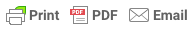Sorry, you do not have permission to ask a question, You must login to ask question. Become VIP Member

Fluid pressure and pascal’s law are explained briefly by considering a small fluid element that is in the state of rest. The concept is more elaborated with the help of a workout example.

## Fluid Pressure at a Point

The fluid pressure acting at a point can be given by the formula:

#### p =F/A;

Where F is the force acting on the fluid over an area A.

Considering a small area dA in the fluid. The force acting on the area dA of this fluid in the state of rest .is in a direction perpendicular to the surface dA. If, dF is the force acting perpendicular to the surface of dA, then pressure acting on a point in a fluid at rest is given by,

p = dF/dA

### Units of Fluid Pressure

The fluid pressure can be measured either in M.K.S units or in S.I Units. In M.K.S units, pressure is measured in kgF/cm2 or kgF/m2. In S.I Units, the fluid pressure is measured in N/mm2 or N/m2.

Note: 1N/m2 = 1Pa; 1bar Fluid Pressure = 100kPa = 100000Pa = 100000N/m2

## What is Pascal’s Law?

Pascal’s law states that the intensity of pressure at a point in a static fluid is equal in all the directions (x,y and z directions). This is proved by the following derivation.& Ad Free!

GET VIP MEMBERSHIP NOW!

Consider an arbitrary fluid element ABC with sides AC, AB and BC as dx,dy, and ds. Here, the width of the element is taken as unity.

Let Px, Py and Pz be the pressure intensities acting on the surfaces AB,AC, and BC. Let the angle ABC be ‘Q’. The whole fluid system is illustrated in the figure-1 above

The forces acting on the fluid element are:

1. The pressure forces on the faces of the element
2. The weight of the whole fluid element acting in the vertical direction downwards.

### 1. Pressure Forces Acting on the Element

Force Acting on AB = Px . Area of the face AB = Px . dy . 1

Force Acting on AC = Py . Area of the face AC = Px . dx . 1

Force Acting on BC = Pz . Area of the face BC = Pz . ds . 1

### 2. Weight of the fluid element

Weight of the fluid element is given by,

w = Mass of fluid element x g = ( Volume x density of fluid ) g

w = [( AB x AC)/2] . 1 . Density of fluid . g

By resolving the forces in,

X-direction

=> Px . dy.1 – Pz.ds.1.sin(90-Q) = 0;

=> Px . dy.1 – Pz.ds.cos Q = 0; [ From figure-1, ds. cos(Q) = dy]

=> Px =Py; (Eq.2)

Y- direction

Py.dx.1 -Pz.ds.1.cos(90-Q) – [ (dx.dy)/2].1.density.g = 0

here also, cos(90-Q) = sinQ and the weight is neglected as the element is very small. Hence we get,

Py =Pz; (Eq.3)

From equation 2 and 3 it is proved that,

Px =Py=Pz

The above equation states that the pressure at a point on a fluid in rest are equal in all direction.

### Workout Example

Consider a hydraulic press with a ram of 0.3m diameter and a plunger of 4.5cm diameter. If the force applied at the plunger is 500N, determine the weight lifted by the hydraulic press.

Solution: Given Data

1. Diameter of Ram = 0.3m
2. Diameter of Plunger = 0.045m
3. Force on Plunger , F = 500N
4. Weight Lifted = ?

From the figure-2, a force is applied at the plunger in order to lift a weight W at the other end of the hydraulic press. The system here follows pascal’s law. As per the law, the stress created at the point of force applied ( At point A) is equal to the stress created at point B. Then,

1. Stress at point A = Pa = Force / Area;

=> Pa = Force / Plunger Area = 500/[(3.124 x .045 x .045)/4] = 314465.4N/m2

2. Pressure Intensity at B = Pb = Force/ Area of Ram;

=> Pb = Pa ( As per pascal’s Law)

=> Pb = 314465.4 = Force/Area of Ram;

Force or Weight lifted = 314465.4 x Area of Ram

= 314465.4 x [(3.14 x 0.3 x 0.3)/4]

Force or Weight lifted = 22222N = 22.2kN